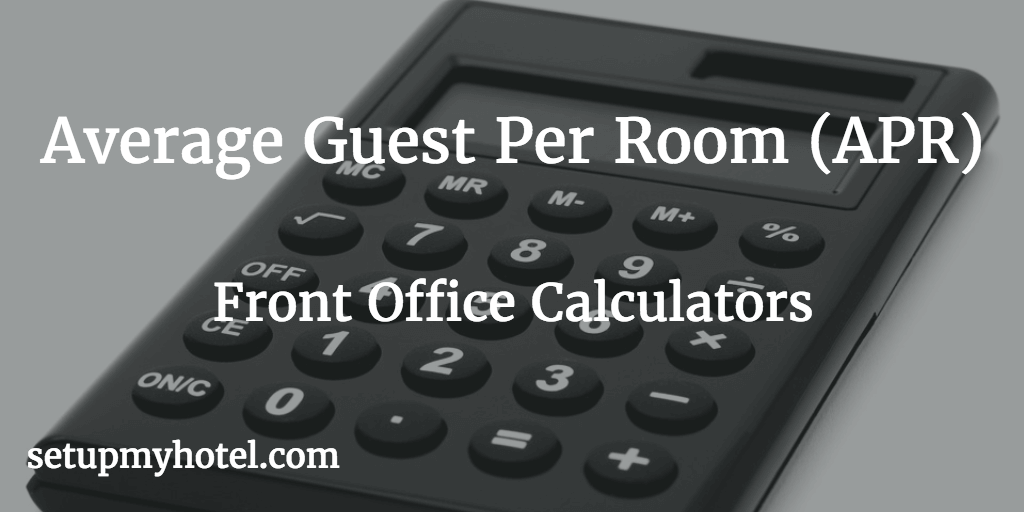# Formula For Average Guest Per Room (APR) - APR Calculator

Average Guest Per Room (APR) - Provides the average number of guests occupied per room in the hotel, This ratio is normally based on the total guest in the hotel including children divided by the total number of rooms sold.

The formula for calculating Average Guest Per Room (APR)

Average Guest Per Room = Total Number of Guests / Number of Rooms Sold

Average Guest Per Room W/O Child = Total Number of Adults / Number of Rooms Sold

Average Rate Per Guest (AGR) Calculator:

Total Room Revenue :

Total No. Of Guests:

Total No. Of Child:

AGR with Total Guests =

AGR Without (W/O) Child =

Example 1 APR with Total Guests:

Total Guest Occupied for 12th September 2017 = 453

Total Rooms Sold for 12th September 2017      = 200

Average Guest Per Room =  453 / 200

= 2.26

Example 2 APR Without (W/O) Child:

Total Adults Occupied for 12th September 2017 = 410

Total Rooms Sold for 12th September 2017       = 200

Average Guest Per Room = 410 / 200

= 2.05

Example 3 APR with Total Guests (Monthly):

Total Guest Occupied for September 2017 = 11935

Total Rooms Sold for September 2017       = 5840

Average Guest Per Room =  11935 / 5840

= 2.04

Example 4 APR Without (W/O) Child (Monthly):

Total Adults Occupied for September 2017 = 11100

Total Rooms Sold for September 2017       = 5840

Average Guest Per Room = 11100 / 5840

= 1.9